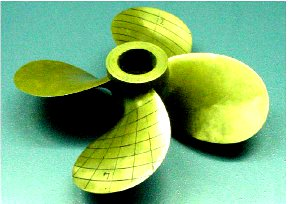# Numerical Prediction of the Cavitating Flow Around Model Scale Propellers by CFD and Advanced Model Calibration

Author(s):
Mitja Morgut, Enrico Nobile (University of Trieste)

Cavitating flows, which can occur in a variety of practical applications, can be modelled using a wide range of methods. One strategy consists of using the RANS (Reynolds Averaged Navier Stokes) approach along with an additional transport equation for the liquid volume fraction, where mass transfer rate due to cavitation is modelled by a mass transfer model.In this study, we verify the influence of three widespread mass transfer models, mainly on the numerical predictions of the propeller performances. The models in question share the common feature of employing some empirical coefficients to tune the models of condensation and evaporation processes, which can influence the accuracy and stability of the numerical predictions. For this reason, and for a fair and congruent comparison, the empirical coefficients of the different mass transfer models are first equally well tuned using an optimization strategy. In this study, we investigated the influence of the mass transfer model on the numerical prediction of the model scale propeller performances. To this aim, we compared three widespread mass transfer models available in the literature. For a fair and congruent comparison, the three different models were equally well tuned by means of an optimization strategy, driven by the modeFRONTIER optimization framework.